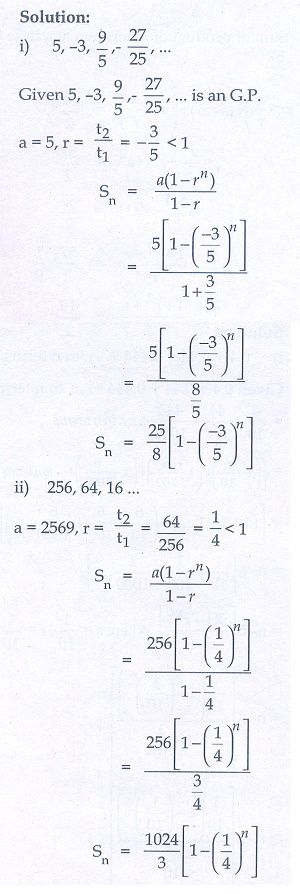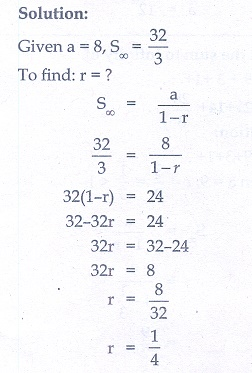Home | | Maths 10th Std | Exercise 2.8: Sum to n/infinite terms of a G.P.(Geometric Progression)

# Exercise 2.8: Sum to n/infinite terms of a G.P.(Geometric Progression)

Maths Book back answers and solution for Exercise questions - Mathematics : Numbers and Sequences: Sum to n/infinite terms of a G.P.(Geometric Progression): Exercise Problem Questions with Answer

### Exercise 2.8

1. Find the sum of first n terms of the G.P. (i) 5,- 3, 9/5 ,- 27/25 ,... (ii) 256,64,16,…2. Find the sum of first six terms of the G.P. 5, 15, 45, …3. Find the first term of the G.P. whose common ratio 5 and whose sum to first 6 terms is 46872.4. Find the sum to infinity of (i) 9 + 3 + 1 +(ii) 21 + 14 + 28/3 + ….5. If the first term of an infinite G.P. is 8 and its sum to infinity is 32/3 then find the common ratio.6. Find the sum to n terms of the series

(i) 0.4 + 0.44 + 0.444 +  to n terms

(ii) 3 + 33 + 333 +   to n terms7. Find the sum of the Geometric series 3 + 6 + 12 +  + 1536 .8. Kumar writes a letter to four of his friends. He asks each one of them to copy the letter and mail to four different persons with the instruction that they continue the process similarly. Assuming that the process is unaltered and it costs ₹2 to mail one letter, find the amount spent on postage when 8th set of letters is mailed.9. Find the rational form of the number.10. If S n  = (x + y ) + (x 2  + xy + y 2 ) + (x 3  + x 2y + xy2  + y 3 ) +  n terms then prove that